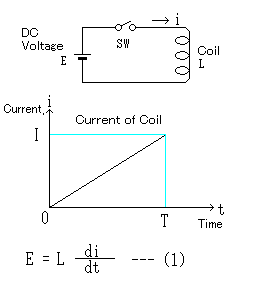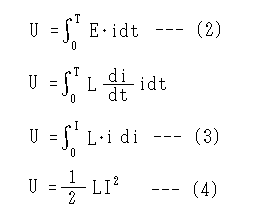﻿

# Energy accumulated in coil

## Is energy accumulated in the coil?

I think that I understand in the person that being able to save the electrical energy has the knowledge of electricity in the capacitor. By the way, the coil Can the electrical energy be saved in (inductor)?

The electrical energy cannot be accumulated like the capacitor of course for a long time. Where did the electrical energy go because the coil did not consume the electrical energy when a pure coil's passing an electric current?It is so, and accumulated in the coil.

## Calculation of energy temporarily saved in coil

### Current when direct voltage is multiplied by coilThe current when the direct voltage is multiplied by the coil is shown in left figure. (1)The direct voltage like the expression The inductance of   E [V] and the coil By   Lenz's law when assuming L [H] Di/dt reaches a constant value.

The increasing rate increases constantly as for the current of the coil at time when switching on by 0 in time. In a word, The T current : I [A] It becomes it. Of course, the resistance of the coil increases and the current increases to infinity if time passes if it is 0 and the power supply is a constant-voltage source of the ideal.

As for a usual coil, there is resistance, and the current of the coil reaches the ceiling because there is internal resistance in a usual power supply.

(1)The expression   Di/dt : Because it is equal to   I/T

E = LI/T     I = It   becomes ET/L.

When the saw wave is thrown into the coil, this expression is used. It used it to calculate the horizontal inclination current of the television of the cathode-ray tube type.

### Calculation of energy accumulated in coilBecause the flowing electrical energy is not consumed anywhere, this energy It becomes energy that U [J] is accumulated in the coil. This becomes the one that a momentary electric power was integrated like the expression (2) at time.

(2)If the expression (1) is substituted and calculated, it becomes an expression like the expression (3) and (4).

In a word, energy U accumulated in the coil.

U = L I 2/ 2

It becomes it. Here, if L is constant, the energy accumulated in the coil is proportion to the second power of the current that flowed at that time.

## Method and problem that accumulates a lot of energy in coil

It is either whether in view of the expression (4), to enlarge the inductance of the coil to increase the energy accumulated in the coil or to increase the current of the coil.

When the coil with a general magnetic substance is passed an electric current, and this current is increased, the magnetic substance causes DC magnetic saturation. Then, the value of the inductance of the coil (L value) becomes small. Therefore, the energy accumulated in the coil reaches the ceiling. Moreover, inductance becomes small if it does so though the gap might be installed in the core so as not to cause DC magnetic saturation.

Being able to do nothing but being to enlarge the magnetic substance inevitably to increase the energy accumulated in the coil is a fact.

## Application of energy accumulated in coil

When energy is accumulated in the coil, is it useful ..what..?

• The resonance happens when the coil is connected with the capacitor and the energy of the coil and the energy of the capacitor are exchanged. This is used as a series resonance and a parallel resonance.
• When the current that flows to the coil is suddenly cut, the energy accumulated in the coil loses the place to go. A high voltage is generated by Lenz's law in the coil. This is used for the igniter of the engine of the car and the blitz device in the electrified fence, etc.
• If the current of the coil enters, is cut similarly, and the coil is united by the core etc. with the coil, the transformer can be made. It is used to raise and lower the voltage with the switching regulator.
• It seems to research the method of storing the electric power to the superconductive coil with various research laboratories seriously. Because there is no moving part if it is possible to achieve it and the response is fast, it is epoch-making though it is very difficult. SMES (Superconducting Magnetic Energy Storage).
• In addition, the thing using the coil uses the energy accumulated in the coil in some shape.